# Quick sort in Data Structures

21 September, 2018

Ratings

## Quick sort

The following are the steps to set up Quick Sort in Data Structures.

1. Select first moment of Array A(or sub array) as pivot.
2. Initiative and J to first and last elements of the array respectively.
3. Increment     :    until  A[i]>pivot;stop
4. Decrement :     until A[j]<pivot,stop
5. if i<j     : change A[i] and A[j]
6. Repeat statements 3,4 and 5 until i>j, i.e when i and j cross each other.
7. Exchange the pivot element with the element pointed to by J which is correct place for pivot

Note that in step 7, The swapping keeps the larger element to the right and smaller element to the left, relative to the pivot.     Example: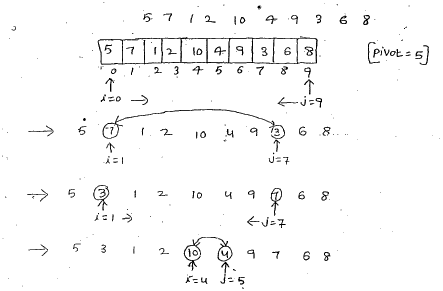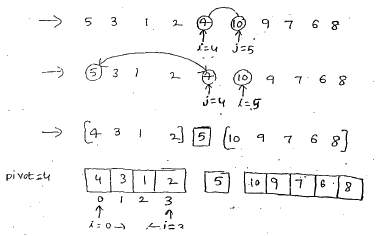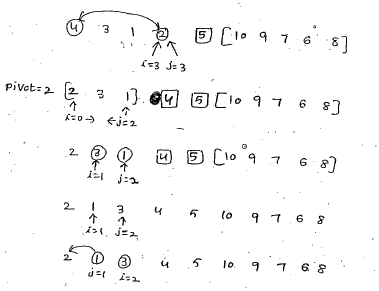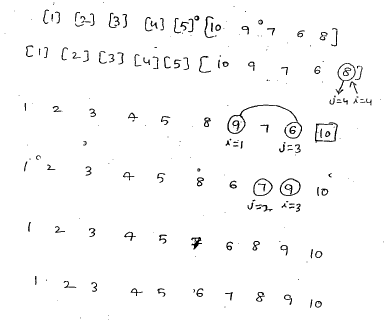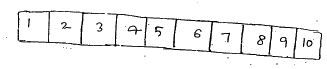### Quick Sort Program

Implementation of Quick sort # include<stdio.h> void Quick sort(int k[], int lb,int vb); void main() { int a; int n,i; clrscr(); printf(“How many numbers:”); scanf(“%d”, &n); printf(“\n Enter the numbers: \n”); for(i=0;i<n;i++) { scanf(“%d”,a[i]); } quicksort(a,o,n-1); printf(“The following are the sorted elements :\n”); for(i=0;i<n;i++) { printf(“%d”,a[i]); } getch(); } void quick sort(int k[], int lb, int vb) { int flag; int i,j,key; flag=1; if(lb<ub) { int temp; i=lb+1; j=ub; key=k[lb];   while(flag) { while(k[i]<key     &&    i<ub) i=i+1; While(k[j]>key    &&    j>lb) j=j-1; if(i<j) { temp=k[j]; k[j]=k[i]; k[i]=temp; } else flag=0; } temp=k[lb]; k[lb]=k[j]; k[j]=temp; quicksort(k,lb,j-1); quicksort(k,j+1,ub); } }

### Output:

How many numbers : Enter the numbers:

### Time complexity:

The running time of sorting algorithm is usually measured by the number f(n) of comparison required to sort ‘n’ elements, the quick sort algorithm which has many variations as been studied extensively generally speaking, The algorithm has a worst case running time of order n2/2=o(n2) it’s an average cape running time of order o(nlogn)